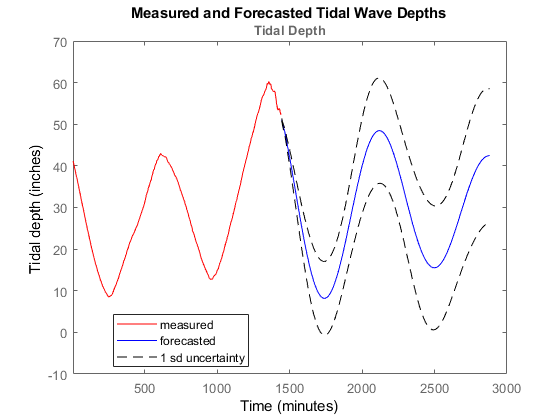# Forecast Tidal Depths Using ThingSpeak Data

This example shows how to forecast the tidal depth at Ockway Bay using data stored in a ThingSpeak™ channel. Predicting tidal depths is vital: if you are not aware of the water depth, your boat can get easily stuck in the mud in a shallow bay.

### Read Data from the Ockway Bay Real-Time Tide Gauge

ThingSpeak™ channel 50289 contains data about tidal depth at Ockway Bay. The data is collected once every 5 minutes. Field 1 of the channel contains tidal depth data. Read the data using the `thingSpeakRead` function from channel 50289 on a particular day, for example, July 01, 2016.

```startDate = datetime('July 1, 2016 12:01:00 AM'); endDate = datetime('July 2, 2016 12:02:00 AM'); dateRange = startDate:endDate; data = thingSpeakRead(50289,'DateRange',dateRange,'Fields',1); ```

### Fit an AR Model to the Data

Use the `iddata` function to create an iddata object of the tidal depth data. Use `detrend` on the data to make the data zero mean. Since the tidal depth varies with time, use the `ar` function to fit a discrete-time autoregressive model to the data. Capture the offset before detrending, and then fit an AR model to represent the system.

```sampleTime = 5; IDdata = iddata(data,[],sampleTime,'OutputName',{'Tidal Depth'},'TimeUnit','minutes') trend = getTrend(IDdata,0); IDdata = detrend(IDdata,0); modelOrder = 8; sys = ar(IDdata,modelOrder); ```
```IDdata = Time domain data set with 288 samples. Sample time: 5 minutes Outputs Unit (if specified) Tidal Depth ```

### Forecast the Tidal Depth

Use the `forecast` function to forecast the tidal depth for the next day. Set the number of samples of the forecast data to be 288 as the measured tidal depth data is updated once every 5 minutes. `yf` is the forecasted model response, and `yf_sd` is the standard deviation of the output. Retrend data before plotting.

```numSamples = 288; [yf,x0,sysf,yf_sd,x,x_sd] = forecast(sys,IDdata,numSamples); IDdata = retrend(IDdata,trend); yf = retrend(yf,trend); ```

### Plot the Forecasted Response

Plot the measured data along with the forecasted tidal data. Also plot the one standard deviation of uncertainties of the forecasted model.

```figure; UpperBound = iddata(yf.OutputData+1*yf_sd,[],yf.Ts,'Tstart',yf.Tstart,'TimeUnit','minutes'); LowerBound = iddata(yf.OutputData-1*yf_sd,[],yf.Ts,'Tstart',yf.Tstart,'TimeUnit','minutes'); plot(IDdata(:,:,[]),'r',yf(:,:,[]),'b'); hold on plot(UpperBound,'k--',LowerBound,'k--'); legend({'measured','forecasted','1 sd uncertainty'},'Location','best'); xlabel('Time'); ylabel('Tidal depth (inches)'); title('Measured and Forecasted Tidal Wave Depths'); ```This plot shows the measured and forecast system response along with one standard deviation uncertainty bounds.